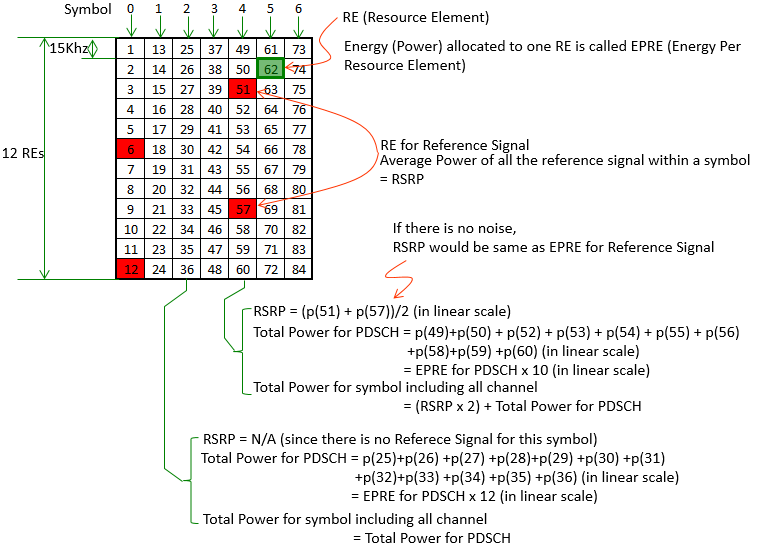LTE Quick Reference RSRP, EPRE, Total Power   We often use different concept of power definition depending on situations and we often get confused by these different power concept. So it is important to have clear understanding on those different definition. When you use various test equipment, there are cases where different equipment use different power concept. For example, some equipment would request you to specify the power in EPRE (power/15 Khz) and some equipment would request you to specify the total power (total power across all the allocated RBs).   Some of the most confusing power concepts are RSRP, EPRE and total power. Definition and Differences among these powers can be illustrated as follows. For the simplicity, I use the structure of only one RB and TM1 (Single Antenna)Directly or indirectly from this illustrations, you can infer some additional facts as follows : EPRE indicate power for one resource element (RE). This can be used for any channel (e.g, Reference Signal, PDSCH etc). This value does not vary with system bandwidth or number of RBs. RSRP is an averaged value for all the Resource Elements for Reference Signal within a symbol. Since this is the averaged value, the value would be similar to EPRE value you set for the Reference Signal. If there is no noise at all, RSRP would be same as EPRE you set for Reference Signal. Total Channel Power is summed value of all EPREs within a symbol. This value may vary with different symbols since each symbol may have different channel combination (e.g, Symbol 0 in first slot is made up of multiple component - PCFICH, HICH, RS. Symbol 4 is made up of PDSCH and Reference signal). For simplicity, if we take the symbol which is made up of only PDSCH (e.g, Symbol 3,5,6) we may come out with the following formula. For different symbols, you may have a little bit different values depending on P-a, P-b configurations. But you can apply this formula for other symbols if you can tolerate around +/- 1dB differences. Total Power of PDSCH (in linear scale ) = EPRE for PDSCH x Number of PDSCH RE = EPRE for PDSCH x Number of RB x 12 (assuming for the symbol with no Reference Signal)   Total Power of PDSCH (in dB/dBm scale ) = EPRE for PDSCH (in dBm) + 10 Log(Number of PDSCH RE) = EPRE for PDSCH + 10 Log(Number of RB x 12)   Total Power is not affected by the system bandwidth, it is affected by number of RBs being used at the specific moment of the calculation.   For example, if you allocated -90 dBm/EPRE for PDSCH and allocated 100 RBs for the PDSCH, the Total Power of PDSCH become as follows. Total Power of PDSCH (in dB/dBm scale ) = EPRE for PDSCH + 10 Log(Number of RB x 12) = -90 + 10 Log(100 x 12) = -90 + 30.8 = - 59.2 dBm